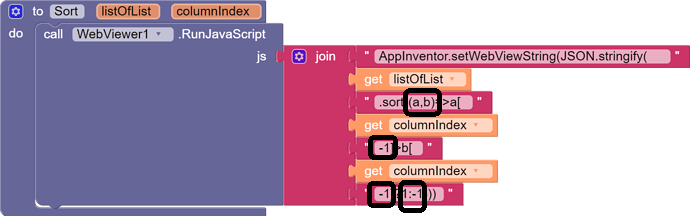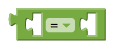# Sorting Tables Ascending and Descending by column contents using Merge Sort

It took me a while to figure out the magic in @Kevinkun's code.Here is my interpretation of the parts:

• the text following .sort is an inline definition of the procedure that compares two rows (a and b) of the input Array.
• (a,b) is the parameter definition part of the procedure definition
• Subtraction of 1 from each columnIndex is necessary because JavaScript indices start at 0, vs AI2 indices which start at 1
• the 1:-1 part is part if an if/then construct (without the "If" and "then" words that applies a test and returns 1 if true, and -1 if false. The test is the comparison of a(columnIndex-1) vs b(columnIndex-1)
• The 1 vs -1 values resulting from the comparison are part of the definition of how the JavaScript .sort feature works. It looks for those values to decide whether or not to swap a and b in the sort. (I don't see the third possible value, 0, here, so that opens the question as to whether or not this setup will preserve the order of list items that did not need to move (= values).

Am I right?

P.S. I did not delve into the definition of the '>' comparison operator in JavaScript.
AI2 has at least 3 of them, in blue, green, and red.

1 Like

do you mean math, logic, and text?
but logic do not have < (less than).

Right, I extrapolated from my memory of the logic '=' block.
I stand corrected.

### = {#=}Tests whether its arguments are equal.

• Two numbers are equal if they are numerically equal, for example, 1 is equal to 1.0.
• Two text blocks are equal if they have the same characters in the same order, with the same case. For example, banana is not equal to Banana.
• Numbers and text are equal if the number is numerically equal to a number that would be printed with that text. For example, 12.0 is equal to the result of joining the first character of 1A to the last character of Teafor2.
• Two lists are equal if they have the same number of elements and the corresponding elements are equal.

Acts exactly the same as the = block found in Math

Hi,
The code is sorting the table but the returning value is not a list. How can I convert it in a table of lists. Please help. Thanks

I am guessing the JavaScript returned the JSON text representation of the list or table.

The Web component has a block to take a JSON text and convert it into dictionaries and lists.